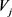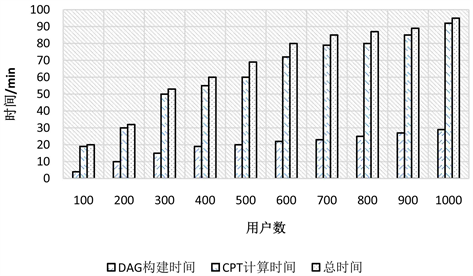# 基于贝叶斯网络的用户行为相似性研究Research on Users Behavior Similarity Based on Bayesian Network

DOI: 10.12677/SEA.2019.82008, PDF, HTML, XML, 下载: 385  浏览: 1,330

Abstract: With the rapid development of mobile devices and mobile services, mobile social networks are integrated into people’s daily lives, and people are also generating a large amount of data here. The research on this huge data source is very meaningful and necessary. User similarity in social networks is an important research field in social media data analysis. It also plays a very important role in the research of product recommendation and social network user relationship evolution. The similarity between users depends not only on the network topology, but also on the degree of dependence between users. In order to achieve the similarity measure between users in social network data, this paper proposes a basis based on topology and probabilistic reasoning. The user similarity measurement method of social network is adopted, and Bayesian network is used as the framework of this uncertain knowledge discovery. A user similarity discovery method based on Bayesian network is proposed.

1. 前言

1.1. 研究背景

1.2. 国内外研究现状

2. 社交网络结构基本特征

2.1. 社交网络的理论基础

2.2. 社交网络的组成元素

2.3. 复杂网络节点相关度(Node-Relevance)

${R}_{ij}={R}_{ji}=\frac{{X}_{i}\cdot {X}_{j}}{|{X}_{i}|\cdot |{X}_{j}|}$

2.4. 贝叶斯网络介绍

1) 存在一个变量集 $V=\left\{{X}_{i}\right\},i=1,2,\cdots ,n$ ，以及变量对应结点之间有向边的集合 $E$

2) 每一个变量都取有限个离散值。

3) 由变量对应的结点和结点之间的有向边构成一个有向无环图 

4) 对于每个结点 ${X}_{i}$ 和它的父结点集 ${\Pi }_{i}$ ，都对应一个条件概率分布表 $p\left({x}_{i}|{\text{π}}_{i},G\right)$ ，而且满足

$p\left({x}_{1},\cdots ,{x}_{n}\right)=\underset{i=1}{\overset{n}{\prod }}p\left({x}_{i}|{\text{π}}_{i},G\right)$

2.5. 贝叶斯网络推理

3. 基于贝叶斯网络学习的用户相似度建模

3.1. 用户相似度贝叶斯网络模型的定义

$V=\left\{{V}_{1},{V}_{2},\cdots ,{V}_{m}\right\}$$T=\left\{{T}_{1},{T}_{2},\cdots ,{T}_{m}\right\}$ 分别为用户集合(即该社交网络中的所有作者集合)、实物集合(即该社交网络中的论文标题集合)和与实物有交互关系的用户集合，其中 ${T}_{i}=\left\{{T}_{i1},{T}_{i2},\cdots ,{T}_{i{l}_{i}}\right\}$${T}_{ij}\in K,1\le i\le n,1\le j\le {l}_{i}$${T}_{ij}$ 表示属于用户 ${V}_{i}$ 所著的某篇论文，用户 ${V}_{i}$ 的所有文章以一个二元组 ${A}_{i}=〈{V}_{i},{Q}_{i}〉$${Q}_{i}=\left\{{T}_{ij}|i=1,2,\cdots ,{l}_{i}\right\}$ 表示用户与论文之间的关系。

1) ${G}_{B}=\left(V,E\right)$ 为USBN的DAG结构，每个用户对应网络结构的一个节点，用户 $V=\left\{{V}_{1},{V}_{2},\cdots ,{V}_{m}\right\}$${G}_{B}$ 的节点集， ${V}_{i}$ 的取值为1或0，表示用户 ${V}_{i}$ 是否著有文章。E为网络结构中的有向边集合，表示用户间的相似性，若用户节点之间存在相似性，则用有向边 ${V}_{i}\to {V}_{i}$ 表示，并称${V}_{i}$ 的一个父节点， ${V}_{i}$${V}_{j}$ 的一个子节点， ${V}_{i}$ 的所有父节点集合为 $Pa\left({V}_{i}\right)$

2) $W=\left\{p\left({V}_{i}|Pa\left({V}_{i}\right)|{V}_{i}\in V\right)\right\}$ 为网络结构中条件概率分布的集合，由各结点相对应的CPT中的值构成， $p\left({V}_{i}|Pa\left({V}_{i}\right)\right)$ 表示结点 ${V}_{i}$ 在其父结点发生情况下的条件概率，用来表示 $Pa\left({V}_{i}\right)$ 的值对 ${V}_{i}$ 的值的影响。

3.2. 用户相似性贝叶斯网络模型的构建

1) 首先需要确定判断用户是否相似，则能够明确两个用户节点间是否存在边；

2) 当明确用户节点之间存在相似关系时，接下来就需要确定用户节点之间有向边的指向。

$\text{sim}\left({V}_{i},{V}_{j}\right)=N\left({Q}_{i}\cap {Q}_{j}\right)/N\left({Q}_{i}\cup {Q}_{j}\right)$ (3.1)

$L\left({V}_{i}|{V}_{j}\right)$ 表示用户  对用户 ${V}_{i}$ 的影响程度， $L\left({V}_{j}{|V}_{i}\right)$ 表示用户 ${V}_{i}$ 对用户 ${V}_{j}$ 的影响程度，则：

$L\left({V}_{i}|{V}_{j}\right)=N\left({Q}_{i}\cap {Q}_{j}\right)/N\left({Q}_{j}\right)$$L\left({V}_{j}|{V}_{i}\right)=N\left({Q}_{i}\cap {Q}_{j}\right)/N\left({Q}_{i}\right)$ (3.2)

$L\left({V}_{i}|{V}_{j}\right)>L\left({V}_{j}|{V}_{i}\right)$ ，则表示用户 ${V}_{j}$ 对用户 ${V}_{i}$ 的影响程度低于用户 ${V}_{i}$ 对用户 ${V}_{j}$ 的影响程度，则节点之间的边的指向为由 ${V}_{j}$ 指向 ${V}_{i}$

3.3. 基于概率推理的用户相似性贝叶斯网络模型的构建

3.4. USBN模型构建效率测试Figure 1. The construction efficiency diagram of USBNFigure 2. The convergence effect diagram of USBN

3.5. USBN收敛性测试

  中国互联网络信息中心(CNNIC)发布的第42次《中国互联网络发展状况统计报告》[R]. http://www.cnnic.net.cn/.  Pearl, J. (1988) Probabilistic Reasoning in Intelligent Systems: Networks of Plausible Inference. Morgan Kaufmann.  Russell, S.J. and Norvig, P. (2010) Artificial Intelligence: A Modern Approach. Applied Mechanics & Materials, 263, 2829-2833.  方滨兴, 贾焰, 韩毅. 社交网络分析核心科学问题, 研究现状及未来展望[J]. 中国科学院院刊, 2015(2): 187-199.  徐娟. 基于贝叶斯网的社交网络用户相似性发现[D]: [硕士学位论文]. 昆明: 云南大学, 2015.  李青. 基于MapReduce的广告点击率预测系统设计与实现[D]: [硕士学位论文]. 昆明: 云南大学, 2016.  郭俊. 大规模复杂网络社区发现与社区进化分析技术研究[D]: [硕士学位论文]. 成都: 西南交通大学, 2017.  方志鹏. 基于贝叶斯网的新广告点击率预测[D]: [硕士学位论文]. 昆明: 云南大学, 2015.  Yan, J., Liu, N., Wang, G., et al. (2009) How Much Can Behavioral Targeting Help Online Advertising? Proceed-ings of the 18th International Conference on World Wide Web, Madrid, 20-24 April 2009, 261-270. https://doi.org/10.1145/1526709.1526745  程学旗. 信息网络拓扑结构与内容相关性研究[D]: [博士学位论文]. 北京: 中国科学院研究生院(计算技术研究所), 2006.  胡笑旋, 杨善林, 马溪骏. 面向复杂问题的贝叶斯网建模方法[J]. 系统仿真学报, 2006, 18(11): 3242-3246.  王双成. 贝叶斯网络学习、推理与应用[M]. 上海: 立信会计出版社, 2010.  贝叶斯网引论[M]. 北京: 科学出版社, 2006.  DBLP 数据集[R]. http://dblp.uni-trier.de/xml/.